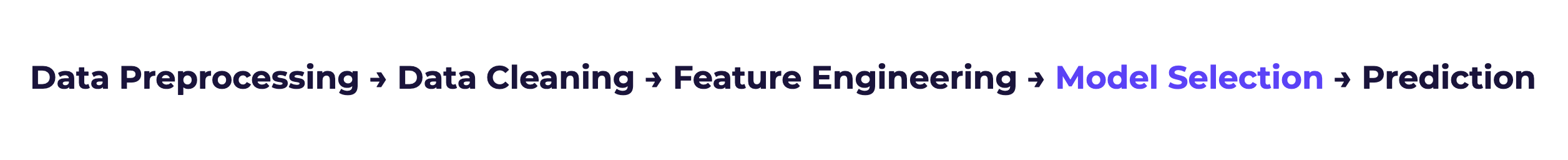# Model Selection

Cortex automatically connects every step of the Machine Learning process into end-to-end Machine Learning Pipelines that anyone in your business can run. In this guide, we’ll discuss the fourth step of a Cortex pipeline: model selection.# What is model selection?

The model selection step is responsible for two things:

1. Training up to hundreds of unique machine learning models, and
2. Staging a competition to find the best one

To train each model, Cortex feeds a set of labeled examples through a particular machine learning algorithm. The algorithm’s job is then to learn a relationship between your pipeline’s features and the example labels that you provided. This relationship (i.e. model) can then be used to make predictions for new data that it hasn’t seen before.

Some of your labeled examples are withheld from training so that they can be used for testing how each model performs on a new set of data. The model whose predictions most closely match the true labels for this holdout set is then chosen as the winner.

For example, in order to predict which users are likely to be homeowners, Cortex would train various models using examples of users whose homeownership status is known. During training, each model would learn which feature patterns tend to be associated with homeowners vs. non-homeowners. The one that predicts homeownership most accurately on a holdout set is selected as the winning model.

# Why does it matter?

There are hundreds of distinct ML algorithms, and each one has many variations (sometimes infinite!). It’s near-impossible to know ahead of time which algorithm is best suited for a given problem. So, the best strategy for achieving high-performance is usually a “trial-and-error” process of building many models and comparing the results. In this sort of head-to-head competition, the cream will rise to the top.

# Which model selection techniques does Cortex use?

### Metalearning

Because of time and computational constraints, it’s never feasible to try every variation of every possible algorithm. But because Cortex takes in continuous streams of data, your pipelines have the opportunity to remember and learn which techniques tend to work well for which problems. This means you’re getting high-performance models without waiting for days on end.

### Algorithm Selection

The below table summarizes the algorithms used for each pipeline type. Cortex trains models using many variations of each algorithm by tuning its hyperparameters (initial conditions of the algorithm set by Cortex before training begins).

 Future Events Uplift Classification Look Alike Regression Recommendations AdaBoost X X X X Artificial Neural Network X X X X X X Collaborative Filtering X Decision Tree X X X X X Gradient Boosted Tree X X X X X Isolation Forest X K-Nearest Neighbors X X X X Linear Regression X Logistic Regression X X X X Random Forest X X X X X Support Vector Machines X X X X X

### Prevention of Overfitting

Overfitting is a common pitfall in machine learning where your model mistakes noise in the training data for a true relationship. A classic sign of overfitting is a model which performs near-perfectly on sample training data, but poorly on new data. Sample techniques for avoiding overfitting include –